# Distribution of critical values

By considering the moments of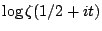, Selberg (in unpublished work) proved that for Borel measurable sets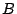,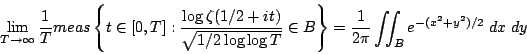which roughly speaking says that, high up the critical line, the real and imaginary parts ofbehave like independent Gaussian random variables with mean zero and variance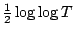.

It is also of interest to look at the tails of this distribution, for example the probability that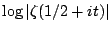takes very large negative values (which will be when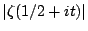is very small). One can make plausible conjectures about the behaviour of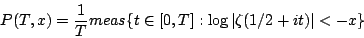when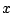is very large, using methods of random matrix theory. In particular, Hughes, Keating and O'Connell [Proc. R. Soc. Lond. 456, 2611--2627] and Keating and Snaith [Comm. Math. Phys 214, 57--89] have conjectured that for large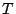,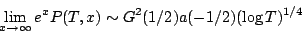where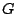is the Barnes-function, and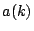is a certain product over primes, coming from mean values of the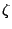-function.

But much more is true (Hughes, PhD thesis). Writing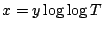, then it is conjectured that for large,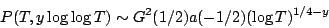uniformly for any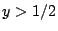. The fact that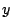is restricted to being greater than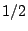is important, since there is a phase transition there: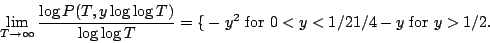And thus we see a change in the behaviour of the left tail of the distribution offrom the Gaussian decay (anticipated from Selberg's result) to exponential decay.

Back to the main index for L-functions and Random Matrix Theory.